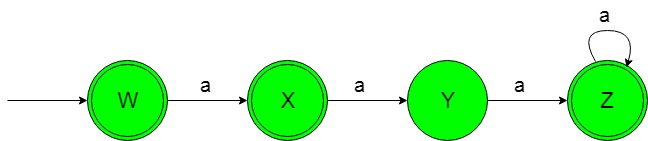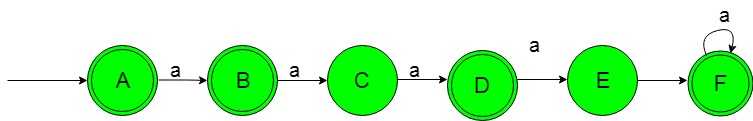GeeksforGeeks App
Open AppBrowser
Continue

# Designing Deterministic Finite Automata (Set 10)

Prerequisite: Designing finite automata
In this article, we will see some designing of Deterministic Finite Automata (DFA).
Problem-1: Construction of a minimal DFA accepting set of strings over {a} in which {an | n≥0, n≠2 i.e, ‘n’ should be greater than 0 and not equal to 2}.
Explanation: The desired language will be like:

`L1 = {ε, a, aaa, aaaa, aaaaa, ..................}`

Here ε is taken as string because value of ‘n’ is greater than or equal to zero and rest of the strings are having ‘a’ to the power of any positive natural number but not 2.
Below language is not accepted by this DFA because some of the string containing ‘a’ to the power 2.

`L2 = {aa, aaaaa, ..........}`

This language L2 is not accepted by this required DFA because of its string containing ‘a’ to the power of 2.
The state transition diagram of the desired language will be like below:In the above DFA, the initial and final state’W’ on getting ‘a’ as the input it transits to a final state ‘X’. The final state ‘X’ on getting ‘a’ as the input it transits to a state ‘Y’. The state ‘Y’ on getting ‘a’ as the input it transits to a final state ‘Z’ which on getting any number of ‘a’ it remains in the state of itself.

## Python3

 `            ` `def` `stateW(n):``    ``#if length of n become 0``    ``#then print accepted``    ``if``(``len``(n)``=``=``0``):``        ``print``(``"string accepted"``)``        ` `    ``else``:``        ``#if at zero index``        ``#'a' found call``        ``#stateX function   ``        ``if` `(n[``0``]``=``=``'a'``):``            ``stateX(n[``1``:])``       ` `            ` `        ` `def` `stateX(n):``    ``#if length of n become 0``    ``#then print accepted``    ``if``(``len``(n)``=``=``0``):``        ``print``(``"string accepted"``)``        ` `    ``else``: ``        ``#if at zero index``        ``#'a' found call``        ``#stateY function   ``        ``if` `(n[``0``]``=``=``'a'``):``            ``stateY(n[``1``:])``        ` `def` `stateY(n):``    ``#if length of n become 0``    ``#then print not accepted``    ``if``(``len``(n)``=``=``0``):``        ``print``(``"string not accepted"``)``        ` `    ``else``:``        ``#if at zero index``        ``#'a' found call``        ``#stateZ function   ``        ``if` `(n[``0``]``=``=``'a'``):``            ``stateZ(n[``1``:])``        ` `def` `stateZ(n):``    ``#if length of n become 0``    ``#then print accepted``    ``if``(``len``(n)``=``=``0``):``        ``print``(``"string accepted"``)``        ` `    ``else``:``        ``#if at zero index``        ``#'a' found call``        ``#stateZ function   ``        ``if` `(n[``0``]``=``=``'a'``):``            ``stateZ(n[``1``:])``            ` `#take input``n``=``input``()` `#call stateW function``#to check the input``stateW(n)`

Problem-2: Construction of a minimal DFA accepting set of strings over {a} in which {an | n≥0, n≠2, n≠4 i.e, ‘n’ should be greater than 0 and not equal to 2 and 4}.
Explanation: The desired language will be like:

`L1 = {ε, a, aa, aaaaa, aaaaaa, .................. }`

Here ε is taken as string because value of ‘n’ is greater than or equal to zero and rest of the strings are having ‘a’ to the power of any positive natural number but not 2 and 4.
Below language is not accepted by this DFA because some of the string containing ‘a’ to the power of 2 and 4.

`L2 = {aa, aaaaa, aaaaaaaaaa, ............. }`

The state transition diagram of the desired language will be like below:In the above DFA, the initial and final state ‘A’ on getting ‘a’ as the input it transits to a final state ‘B’. The final state ‘B’ on getting ‘a’ as the input it transits to a state ‘C’. The state ‘C’ on getting ‘a’ as the input it transits to a final state ‘D’. The final state ‘D’ on getting ‘a’ as the input it transits to a state ‘E’. The state ‘E’ on getting ‘a’ as the input it transits to a final state ‘F’. The final state ‘F’ on getting ‘a’ as the input it remains in the state of itself.

## Python3

 `            ` `def` `stateA(n):``    ``#if length of n become 0``    ``#then print accepted``    ``if``(``len``(n)``=``=``0``):``        ``print``(``"string accepted"``)``        ` `    ``else``:``        ``#if at zero index``        ``#'a' found call``        ``#stateB function   ``        ``if` `(n[``0``]``=``=``'a'``):``            ``stateB(n[``1``:])``       ` `            ` `        ` `def` `stateB(n):``    ``#if length of n become 0``    ``#then print accepted``    ``if``(``len``(n)``=``=``0``):``        ``print``(``"string accepted"``)``        ` `    ``else``: ``        ``#if at zero index``        ``#'a' found call``        ``#stateC function   ``        ``if` `(n[``0``]``=``=``'a'``):``            ``stateC(n[``1``:])``        ` `def` `stateC(n):``    ``#if length of n become 0``    ``#then print not accepted``    ``if``(``len``(n)``=``=``0``):``        ``print``(``"string not accepted"``)``        ` `    ``else``:``        ``#if at zero index``        ``#'a' found call``        ``#stateD function   ``        ``if` `(n[``0``]``=``=``'a'``):``            ``stateD(n[``1``:])``        ` `def` `stateD(n):``    ``#if length of n become 0``    ``#then print accepted``    ``if``(``len``(n)``=``=``0``):``        ``print``(``"string accepted"``)``        ` `    ``else``:``        ``#if at zero index``        ``#'a' found call``        ``#stateE function   ``        ``if` `(n[``0``]``=``=``'a'``):``            ``stateE(n[``1``:])` `def` `stateE(n):``    ``#if length of n become 0``    ``#then print not accepted``    ``if``(``len``(n)``=``=``0``):``        ``print``(``"string not accepted"``)``        ` `    ``else``:``        ``#if at zero index``        ``#'a' found call``        ``#stateF function   ``        ``if` `(n[``0``]``=``=``'a'``):``            ``stateF(n[``1``:])``            ` `def` `stateF(n):``    ``#if length of n become 0``    ``#then print accepted``    ``if``(``len``(n)``=``=``0``):``        ``print``(``"string accepted"``)``        ` `    ``else``:``        ``#if at zero index``        ``#'a' found call``        ``#stateF function   ``        ``if` `(n[``0``]``=``=``'a'``):``            ``stateF(n[``1``:])           ``            ` `#take input``n``=``input``()` `#call stateA function``#to check the input``stateA(n)`

My Personal Notes arrow_drop_up